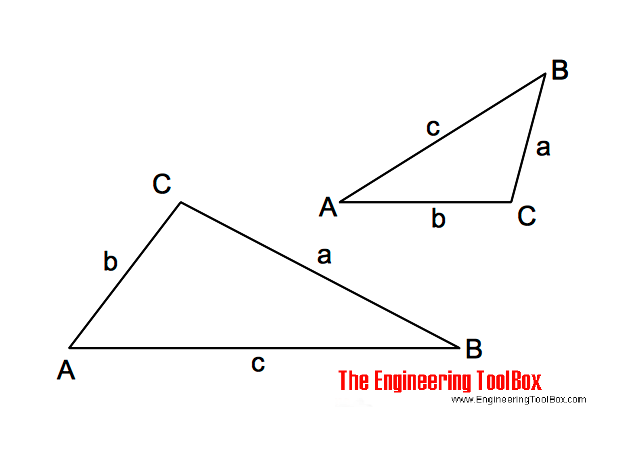Engineering ToolBox - Resources, Tools and Basic Information for Engineering and Design of Technical Applications!

# Oblique Triangle

## Calculate oblique triangles.

An oblique triangle is any triangle that is not a right angled triangle.### Triangle Three Known Values - Length and Angle Calculator

Calculate the unknown lengths and angles in a triangle. Add three known values - leave the rest of the inputs blank.

Note! - the calculator is based on the same value combinations used in the equations below. Other value combinations will not work - most triangles with three known values can be adapted to these equations. The calculator is quite simple. With strange results - check your input values.

Area (m2, mm2, ft2, in2 .....):

(enable pop-up)

### A, B and a is known, calculate

b = a sin(B) / sin(A)                       (1a)

C = 180o - (A + B)                        (1b)

c = a sin(C) / sin(A)                       (1c)

### A, a and b is known, calculate

sin(B) = b sin(A) / a                      (2a)

C = 180o - (A + B)                      (2b)

c = a sin(C) / sin(A)                      (2c)

### a, b and C is known, calculate

tan(A) = a sin(C) / (b - a cos(C))                 (3a)

B= 180o - (A + C)                        (3b)

c = a sin(C) / sin(A)                       (3c)

### a, b and c is known, calculate

cos(A) = (b2 + c2 - a2) / (2 b c)                      (4a)

cos(B)= (a2 + c2 - b2) / (2 a c)                      (4b)

C = 180o - (A + B)                              (4c)

### a, b, c, A, B and C is known, calculate area

s = (a + b + c) / 2                        (5a)

area = (s (s - a) (s- b) (s - c))1/2                     (5b)

area = b c sin(A) / 2                           (5c)

area = a2 sin(B) sin(C) / (2 sin(A))                     (5d)

## Related Topics

• ### Mathematics

Mathematical rules and laws - numbers, areas, volumes, exponents, trigonometric functions and more.

## Related Documents

• ### Algebraic Expressions

Principal algebraic expressions formulas.
• ### Angle Calculator - Carpenter's Square

Calculate angles with a straight board across carpenter's square.
• ### Geometric Shapes - Areas

Areas, diagonals and more - of geometric figures like rectangles, triangles, trapezoids ..
• ### Hyperbolic Functions

Exponential functions related to the hyperbola.
• ### Law of Tangents

Triangles and law of tangents.
• ### Laws of Indices

Simplifying calculations by involving indices.
• ### Miter Saw - Calculate Sawing Angle

Calculate miter saw protractor angles for skirting and decorative mouldings work.
• ### Pythagorean Theorem

Verifying square corners.
• ### Regular Polygons

Areas of regular polygons - polygons with 3 to 12 sides.
• ### Right Angled Triangle

Right angled triangle equations.
• ### Triangle

Triangle analytical geometry.
• ### Trigonometric Functions

Sine, cosine and tangent - the natural trigonometric functions.

Online vector calculator - add vectors with different magnitude and direction - like forces, velocities and more.

## Engineering ToolBox - SketchUp Extension - Online 3D modeling!

Add standard and customized parametric components - like flange beams, lumbers, piping, stairs and more - to your Sketchup model with the Engineering ToolBox - SketchUp Extension - enabled for use with older versions of the amazing SketchUp Make and the newer "up to date" SketchUp Pro . Add the Engineering ToolBox extension to your SketchUp Make/Pro from the Extension Warehouse !

We don't collect information from our users. More about

## Citation

• The Engineering ToolBox (2014). Oblique Triangle. [online] Available at: https://www.engineeringtoolbox.com/oblique-triangle-formulas-d_1871.html [Accessed Day Month Year].

Modify the access date according your visit.

10.2.10